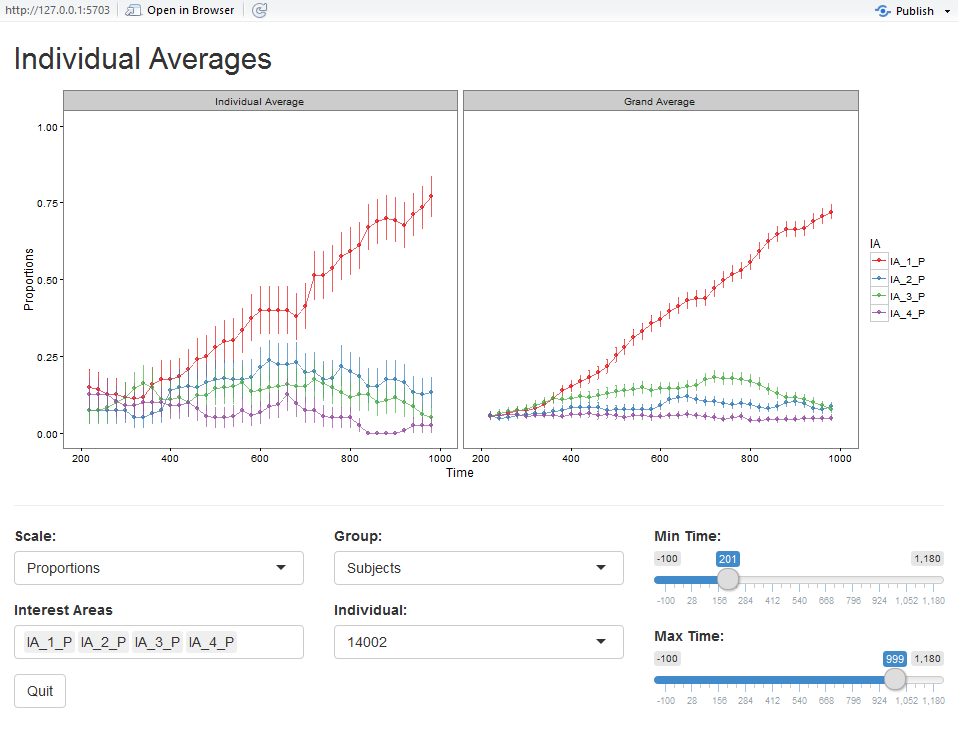# Plotting SR Eyelink Data Processed with VWPre

## Plotting the data

It’s often desirable to visualize the proportion (or empirical logit) data, either as a grand average or by condition. In some cases it is even necessary to visualize the trend in the data over a continuous predictor. So, the functions plot_avg and plot_avg_contour provide straightforward plotting options for such cases. These functions internally calculate the average(s) and plot the results. The plotting is powered by ggplot2, so further customization (plot titles, custom themes, etc) is still possible. For more information about ggplot2, please refer to its reference manual and extensive documentation.

### Grand average

Using the function plot_avg, it is possible to plot the grand average of the data by interest area. The parameter type specifies which type of plot to create: proportion or empirical logit. In IAColumns, list the interest area proportion columns (here we have used the default names) along with desired labels.

plot_avg(data = dat, type = "proportion", xlim = c(0, 1000),
IAColumns = c(IA_1_P = "Target", IA_2_P = "Rhyme", IA_3_P = "OnsetComp",
IA_4_P = "Distractor"),
Condition1 = NA, Condition2 = NA, Cond1Labels = NA, Cond2Labels = NA,
ErrorBar = TRUE, VWPreTheme = TRUE)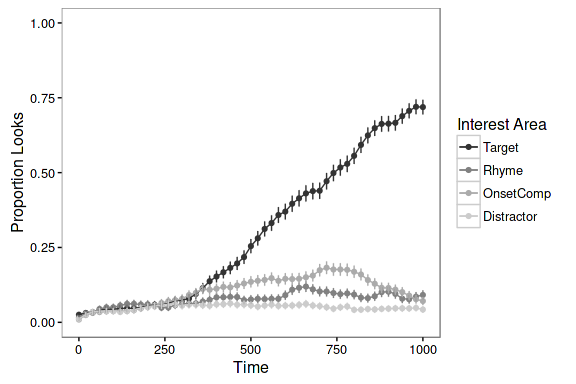To add a title to the plot, simply add the title function from ggplot2.

plot_avg(data = dat, type = "proportion", xlim = c(0, 1000),
IAColumns = c(IA_1_P = "Target", IA_2_P = "Rhyme", IA_3_P = "OnsetComp",
IA_4_P = "Distractor"),
Condition1 = NA, Condition2 = NA, Cond1Labels = NA, Cond2Labels = NA,
ErrorBar = TRUE, VWPreTheme = TRUE) + ggtitle("Grand Average Plot")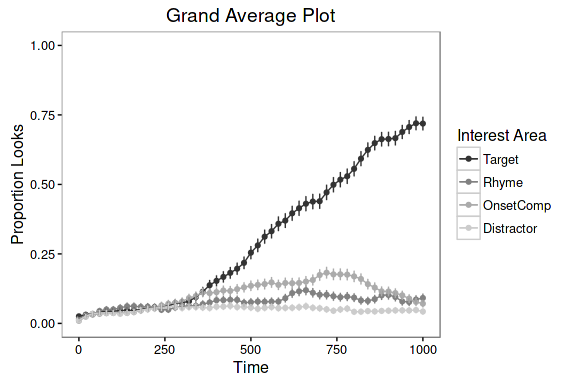To customize the appearance of a plot (e.g., font, size, color, margins, etc.), the VWPreTheme parameter can be set to FALSE, which reverts to the default theming in gglpot2. In this way, the user can apply a custom theme to the plot. Detailed information about creating themes can be found at ggplot2 Theme Vignette. For the purpose of illustration, the default ggplot2 theme has been applied, with the axis text elements increased in size.

plot_avg(data = dat, type = "proportion", xlim = c(0, 1000),
IAColumns = c(IA_1_P = "Target", IA_2_P = "Rhyme", IA_3_P = "OnsetComp",
IA_4_P = "Distractor"),
Condition1 = NA, Condition2 = NA, Cond1Labels = NA, Cond2Labels = NA,
ErrorBar = TRUE, VWPreTheme = FALSE) + theme(axis.text = element_text(size = 15))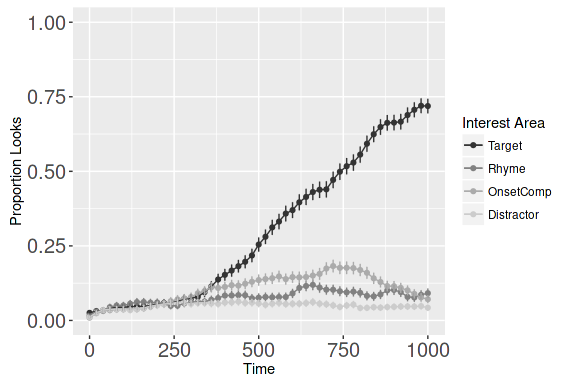### Conditional averages

The function plot_avg can also be used to plot averages for different conditions, based on a factor variable in the data. If the current factor level labels are not suitable for plotting, specify new labels using a list in Cond1Labels.

Specifying Condition1 will stack the plots.

plot_avg(data = dat, type = "proportion", xlim = c(0, 1000),
IAColumns = c(IA_1_P = "Target", IA_2_P = "Rhyme", IA_3_P = "OnsetComp",
IA_4_P = "Distractor"), Condition1 = "talker",
Condition2 = NA, Cond1Labels = c(CH1 = "Chinese 1", CH10 = "Chinese 3",
CH9 = "Chinese 2", EN3 = "English 1"),
Cond2Labels = NA, ErrorBar = TRUE, VWPreTheme = TRUE)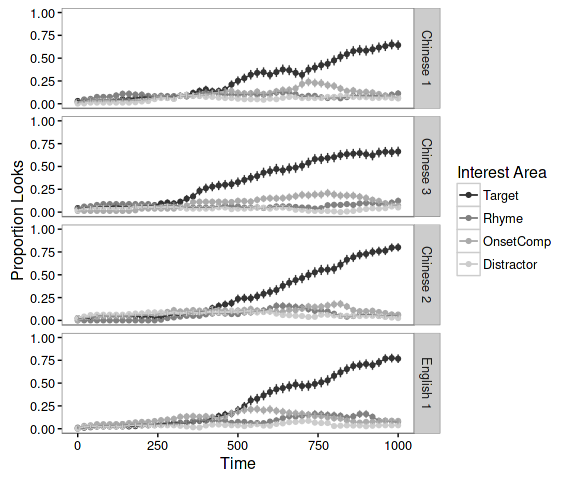Alternatively, specifying just Condition2 will plot the same information, but align it horizontally.

plot_avg(data = dat, type = "proportion", xlim = c(0, 1000),
IAColumns = c(IA_1_P = "Target", IA_2_P = "Rhyme", IA_3_P = "OnsetComp",
IA_4_P = "Distractor"), Condition1 = NA,
Condition2 = "talker", Cond1Labels = NA, Cond2Labels = c(CH1 = "Chinese 1",
CH10 = "Chinese 3",
CH9 = "Chinese 2",
EN3 = "English 1"),
ErrorBar = TRUE, VWPreTheme = TRUE)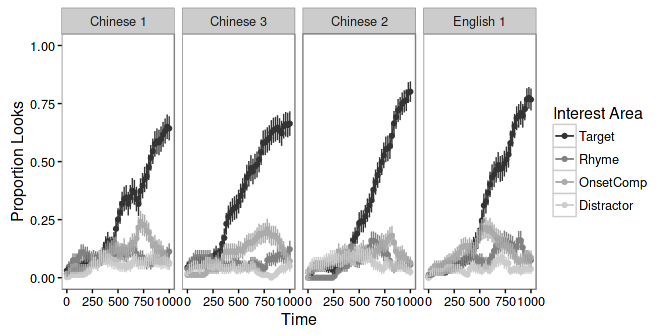For a 2x2 design, it is possible to specify both conditions. This will create a grid-style plot.

plot_avg(data = dat, type = "proportion", xlim = c(0, 1000),
IAColumns = c(IA_1_P = "Target", IA_2_P = "Rhyme", IA_3_P = "OnsetComp",
IA_4_P = "Distractor"), Condition1 = "talker",
Condition2 = "Exp", Cond1Labels = c(CH1 = "Chinese 1", CH10 = "Chinese 3",
CH9 = "Chinese 2", EN3 = "English 1"),
Cond2Labels = c(High = "High Exp", Low = "Low Exp"), ErrorBar = TRUE,
VWPreTheme = TRUE)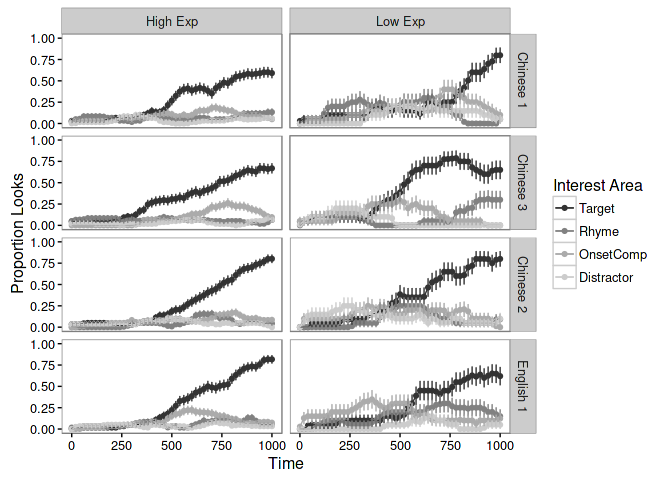### Difference plots

The function plot_avg_diff can also be used to plot the average difference between looks to two interest areas. As with plot_avg upto two conditions can be supplied for conditional plotting.

plot_avg_diff(data = dat, xlim = c(0, 1000), DiffCols = c(IA_1_P = "Target", IA_2_P = "Rhyme"),
Condition1 = NA, Condition2 = NA, Cond1Labels = NA,
Cond2Labels = NA, ErrorBar = TRUE, VWPreTheme = TRUE)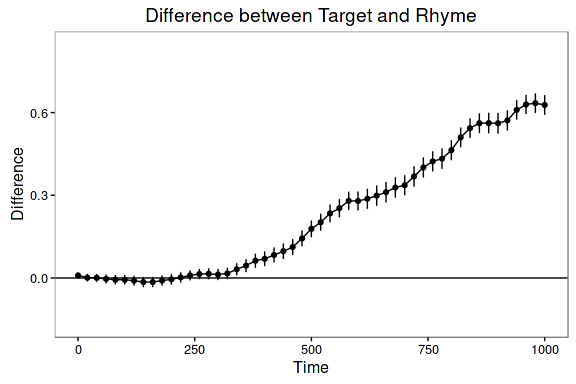plot_avg_diff(data = dat, xlim = c(0, 1000), DiffCols = c(IA_1_P = "Target", IA_2_P = "Rhyme"),
Condition1 = "talker", Condition2 = NA, Cond1Labels = c(CH1 = "Chinese 1",
CH10 = "Chinese 3", CH9 = "Chinese 2", EN3 = "English 1"),
Cond2Labels = NA, ErrorBar = TRUE, VWPreTheme = TRUE)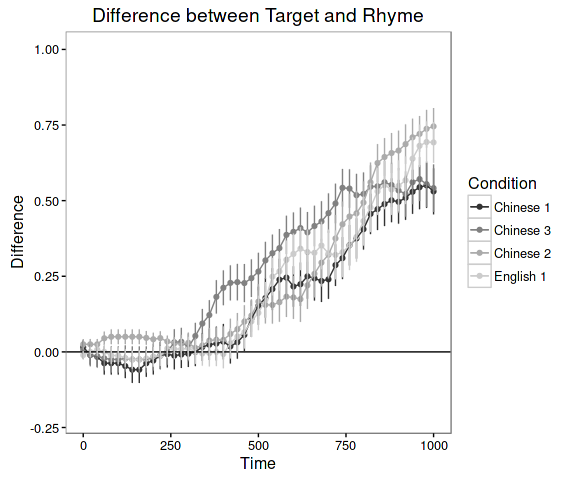### Conditional contour surface

In some cases, studies have not employed a factorial design; rather they aim to investigate continuous variables. Therefore, using the function plot_avg_contour it is also possible to create a contour plot representing the looks to one interest area as a surface over the continuous variable and Time. This function calculates the average time series at each value of the continuous variable and applies a 3D smooth (utilizing gam) over the surface. The function then plots the result as a contour plot. Here, the example plots looks to the target as a function of Rating and Time.

plot_avg_contour(data = dat, IA = "IA_1_P", type = "proportion", Var = "Rating",
VarLabel = "Accent Rating", xlim = c(0,1000), Theme = FALSE,
Color = c("gray20", "gray90"))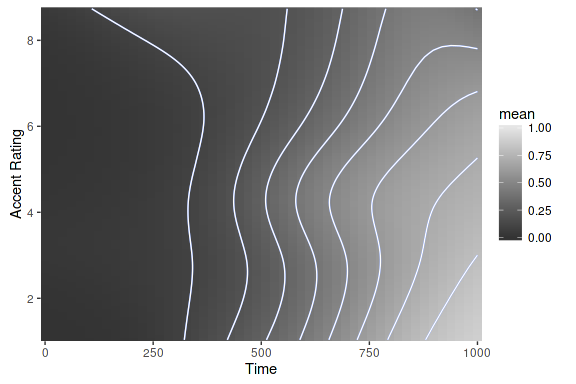It is possible to change the contour colors and add a title. ggplot2 accepts predefined palette colors, RGB, hexadecimal, among others.

plot_avg_contour(data = dat, IA = "IA_1_P", type = "proportion", Var = "Rating",
VarLabel = "Accent Rating", xlim = c(0,1000), Theme = FALSE,
Color = c("red", "green")) + ggtitle("Looks to target")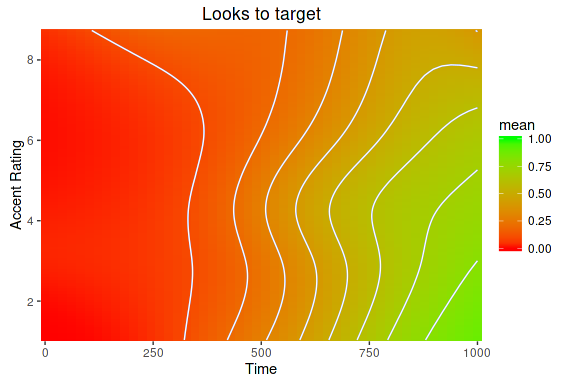## Diagnostic/Utility plots

### Shiny app for understanding the empirical logit transformation

Because users may be inclined to perform an empirical logit transformation on their data, it is important to understand how the transformation works. The transformation converts proportions (which are inherently bound between 0 and 1) to an unbounded measure ranging, in principle, between -Inf and Inf. This presents the issue of the number of observations used in the calculation; this is because the number of samples in the data is inherently linked to the sampling rate at which the recording was done. Therefore, the user may choose to change the number used in the calculation; however, the number of observations changes the shape and range of the transformed values (and their associated weights).

The function plot_transformation_app opens a Shiny App which allows the user to visualize the effect of both number of observations and constant on the result of the empirical logit transformation and weight calculations. These values are plotted against proportions (0 to 1).

plot_transformation_app()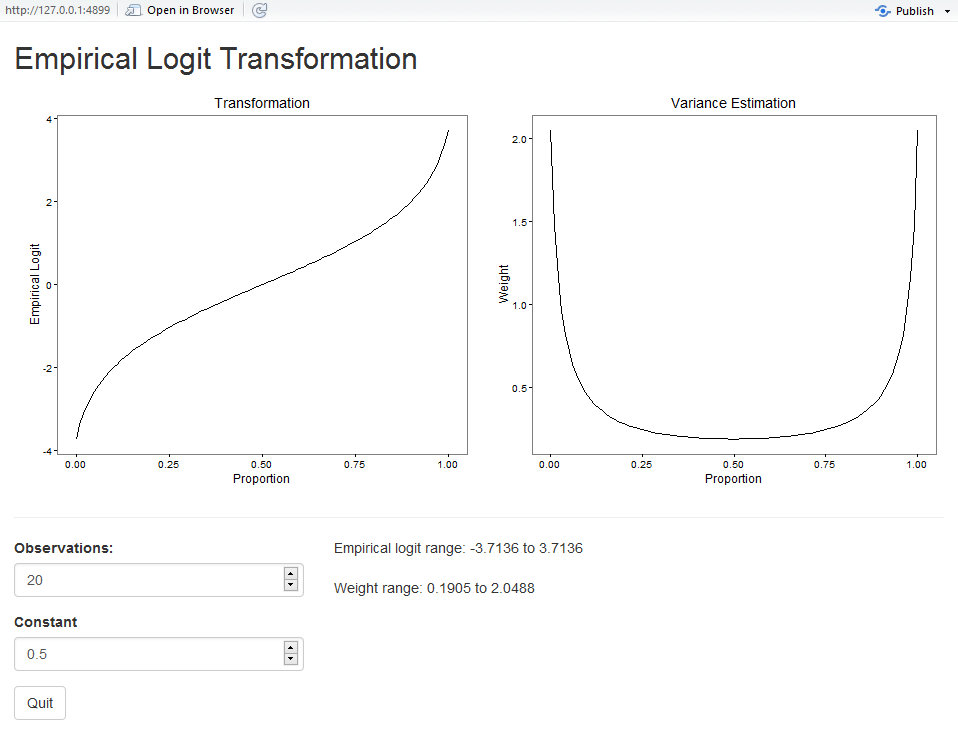### Shiny app plots for data inspection

There are two functions which provide diagnostic Shiny apps for inspecting the data: plot_var_app and plot_indiv_app. These are interactive and allow the user to inspect variability among subjects and items as well as individual averages compared to the grand average. In this way, the user can determine if there are particular subjects or items that differ substantially from the average.

The function plot_var_app allows the user to view by-subject or by-item Z-scores with respect to the overall mean. For this the user provides the desired interest area and time window. The length of the line indicates how far above or below the mean a particular subject or item is within the window. Additionally, the gray circles indicate the SD within each subject or item.

plot_var_app(dat)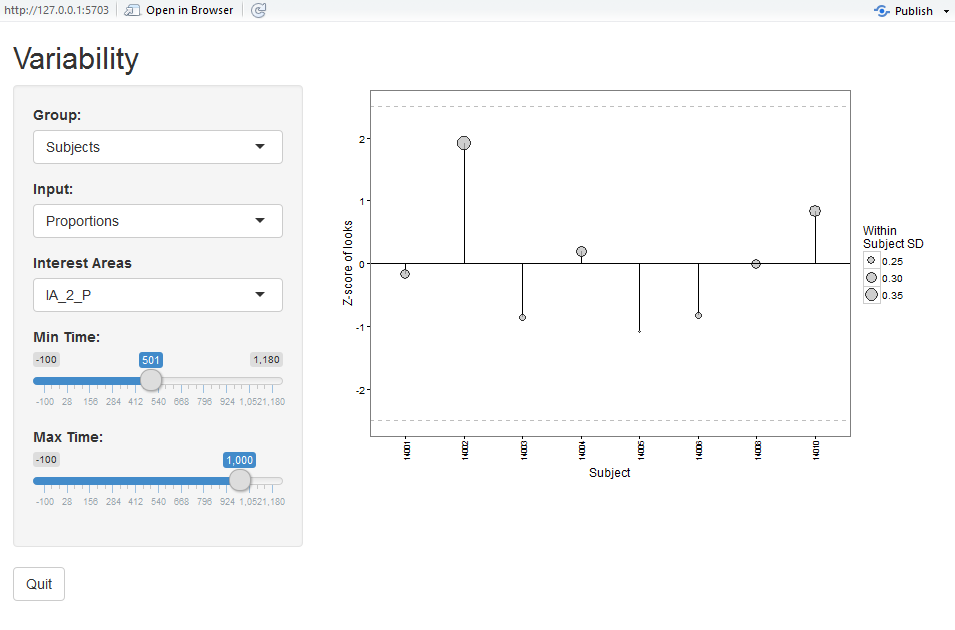The function plot_indiv_app allows the user to view by-subject or by-item averages for all interest areas, along side the grand average. For this the user provides the desired interest areas and time window.

plot_indiv_app(dat)